﻿ MotionDesigner Reference & User Interface > Terminology and Symbols

# Terminology and Symbols

Navigation:  MotionDesigner Reference & User Interface >

# Terminology and Symbols

### MotionDesigner Terminology:

Segments :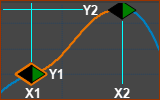A Segment A motion is split into segments. See ab0ve. Each Segments has a duration - called its Segment-Width The Segment-Width is measured on the X-axis. In the image, the Segment-Width is from X1 to a X2. Each Segment has a Motion-Law. The Motion-Law specifies the motion-values between X1,Y1 and X2,Y2. The user specifies the motion-values at X1,Y1 (Start Blend-Point) and X2,Y2 (End Blend-Point).

Blend-Points :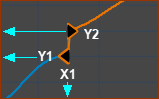A Blend-Point X-Axis: Blend-Points do not have a duration. They have one X-axis value (X1) Y-axis: each Blend-Point has TWO Y-axis motion-values, for each motion-derivative (Y1 and Y2) For each motion-derivative, each Blend-Point has a motion-value at the End of the Previous-Segment (x1,y1) AND at the Start of the Segment (x1,y2). For each motion-derivative, the two motion-values can be different or they can be forced to be the same.

Other Important Terminology

 Motion-Law : You must select a Motion-Law for each Segment. The Motion-Law should be able to satisfy the motion requirements for your design. Each Motion-Law is a mathematical function. The Motion-Law, together with the motion-values at its Blend-Points, specify the motion-values between each Blend-Point. Motion-Derivative : Velocity, Acceleration, and Jerk are the first, second, and third motion-derivatives of Position. Segment Editor : Use the Segment-Editor to edit the Segment-Width, to select the Motion-Law, to edit the Segment-Parameters, and to edit the motion-values at the Start of the Selected-Segment(y1) and at the End of the Selected-Segment(y2). Blend-Point Editor : Use the Blend-Point Editor to edit the X-axis of the Blend-Point (X1) - at the start of the Selected-Segment Edit the motion-values at the End of the Previous-Segment(y1) and at the Start of the Selected-Segment (Y2) Selected-Segment : The active segment. It is a different color to the other segments. Previous-Segment : The segment that precedes (is to the left of / is earlier than / is before) the Selected-Segment. If the Selected-Segment is the first segment, then the Previous-Segment is the last segment. Next-Segment : The segment that follows (is to the right of / is later than / is after) the Selected-Segment. If the Selected-Segment is the last segment, then the Next-Segment is the first segment.

### Blend-Point Symbols

 Each Blend-Point has a one(1) X-axis value. It is the instant on the X-axis when a segment ends and the next segment starts. The symbol for a Blend-Point is the shape of a diamond. The diamond shape is actually two(2) triangles.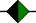Blend-Point - the symbol is two triangles. Each triangle is a symbol for the end of a segment and the start of a segment.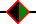Active Blend-Point - has an, outline. It is the Blend-Point at the start of the Selected Segment.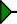The Start of a Segment. The symbol may be Green, Black, Clear, or Grey.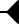The End of a Segment. The symbol may be Black, Clear or Grey. Note: To increase the size of the symbols, see: Active-Motion-Settings > Accessibility > Line and Symbol Sizes > Symbol Size.